# ESS .pdf

### File information

This PDF 1.5 document has been generated by TeX / pdfTeX-1.40.17, and has been sent on pdf-archive.com on 26/05/2017 at 04:37, from IP address 81.153.x.x. The current document download page has been viewed 740 times.
File size: 149.73 KB (11 pages).
Privacy: public file

### Document preview

Viewership Project
Miles Lunn
May 2017

0.1

Introduction

In this project, I aim to test some of the points made in Thorin’s Esports Salon video. For context, I am
mathematics student and have just completed my first year at University. I have completed two modules
based on Statistics, thus I aim to apply them to Esports in this project.

Chapter 1
Nationality &amp; Stream Viewership
1.1

North America

In the video, Noah claimed that NA LCS imports average less viewers than North American players. A
statistical regression line test can be formed to test whether this claim is true. Below summarizes a table
of data for North American Players in the NA LCS:
Player
Contractz
Sneaky
Stixxay
Moon
Hai
Dardoch
Pobelter
Meteos
Stunt
Lourio
Doublelift
WildTurtle

Hours
Streamed/Month
38
132
15
31
32.5
21.5
28
61
48
43
112
72
16

Avg. viewers

Coefficient

AVC

765
13,423
401
267
123
1,005
582
2,702
4,518
66
640
12,607
2,175

1.667
1.842
1
1.339
1
1.704
1.339
1
1.745
1.704
1.745
1.250
1

1,275
24,725
401
357
123
1712
779
2,702
7,885
112
1,117
15,758
2,175

The following players either have streamed League of Legends for less than 10 hours within 30 days.
These players will not be used for the test.
• Smoothie
• Balls
• Altec
• LemonNation
• zig
• LOD
• Keith
• Aphromoo

• Darshan
• Xpecial
• Apollo
• Hakuho
• Matt
• Hauntzer
One factor which will effect player viewer count is the time of day at which they stream. Twitch
usually peaks around 9:30pm, usually holding around 1, 000, 000 viewers  . The minimum viewer count is
around 500, 000, which occurs around 10:00am. In consideration of this, I will multiply the viewer count
by a coefficient for each streamer, depending on typical time of day at which they stream. For example, if
somebody typically streams around 10am, there are 50% of the maximum viewer pool on Twitch at that
time, thus I will multiply their viewer count by 2, to match somebody who streams during peak hours.
AVC stands for adjusted viewer count, which is calculated by multiplying the original viewer count by the
coefficient.
Pearson’s Product Moment Correlation Coefficient can be used to tell us if there is a relation between
monthly hours spent streaming, and viewer count for NA players. This is given by:
P
xy − nxy
r= pP
P
2
[ x − nx2 ][ y 2 − ny 2 ]
Here are some values which are needed to solve the equation for r:
X

xy = 5.2344 × 106
X

X

x2 = 48214

y 2 = 9.40578
x = 50
y = 4548
n = 13

Where x and y are the mean values of x and y respectively.
⇒ r = 0.701

Since r = 0.701, it can be inferred that there is a strong, positive correlation between how often a player
streams on Twitch, and their average viewer count. This means it would be reasonable to produce a linear
regression model: an equation which will tell us how many viewers a North American player should get,
when we are given how often they stream. This equation will be in the form y = a + bx, where a and b
are constants, which are calculated using the formulae:
P
xy − nxy
b= P 2
x − nx2
a = y − bx
⇒ b = 145
⇒ a = −2701
Now we have an equation which tells us roughly how many viewers a North American streamer has,
y , when given the hours they stream per month, x. This equation is: y = 145x − 2701. This is far
from a perfect model, and it only fits specific criteria. For example, if a player streams for less than 18
hours a month, they are given a negative number of viewers. The sample size, n, was very small, and so
extreme results have a large impact on the line equation which has been derived. However, this problem
cannot easily be resolved due to the nature of the NA LCS, which only holds 50 players, many of which
are imports.

1.2

Imports

A second equation must be formed, which can then be compared with the ”North American Player”
equation above. A similar table below has been made for NA LCS imports.
Player
Ray
Xmithie
HuHi
Froggen
Flame
Cody Sun
Olleh
Arrow
Ssumday
Chaser
Keane
lira
Piglet
Svenskeren
Bjergsen
Biofrost

Hours
Streamed/Month
31
30
10
28
28
29
27.5
136
42.5
14
48
11
19
20
13
17.5

Avg. viewers

Coefficient

AVC

1,045
91
178
2,466
48
50
193
208
181
36
161
142
383
2,456
21,525
944

1.745
1.590
1.368
1
1.368
1.675
1.842
1.745
1.704
1.704
1.704
1.745
1.704
1.675
1.842
1

1,824
145
244
2,466
66
84
355
363
308
61
274
248
652
4114
39641
944

The following players either have streamed League of Legends for less than 10 hours within 30 days.
These players will not be used for the test.
• Impact
• Jensen
• Looper
• Gate
• Ryu
• Inori
• Seraph
• Ninja
• Reignover
The 3 imports with the highest viewership are all Danish (Froggen, Svenskeren, Bjergsen). These
players have around 10× as many viewers as non-danish players. I will not include them in my formula
as they will have a very significant impact on the equation.
The Product Moment Correlation Coefficient for the second set of data tells us that r = −0.023. This
implies that there is no correlation between how frequent an imported player streams, and their mean
viewer count. Thus it would be unreasonable to find an equation using the data we have. Because of this,
we cannot compare regression models for North American and Imported players, instead, we will compare
the means of each set of data via hypothesis testing.

1.3

Hypothesis Testing

Two hypotheses will be tested, which are as follows:
H0 : µ1 = µ2
H1 : µ1 6= µ2
Where µ1 is the mean viewers for North American players, for the entire population, and µ2 is the mean
viewers for imported players, for the entire population. The results will therefore account for all future NA
LCS players, as well as present players. H0 is the null hypothesis, claiming that North American players
average the same viewers as imported players. In contrast, H1 is the alternative hypothesis, claiming the
average viewer count for North American and Imported players is not equal. To test this claim, t0 must
be calculated.
x − x2
q1
∼ tn1 +n2 −2
1
1
sp n1 + n2
s
(n1 − 1)s21 + (n2 − 1)s22
sp =
n1 + n2 − 2
P
(x − x)2
s2 =
n−1
t0 =

Where s21 is the sample variance for North American Players, s22 is the sample variance for Imported
players, and s2p is the pooled sample variance. N.B: x denotes the mean adjusted viewer count for this
hypothesis test. It previously denoted monthly hours spent streaming.
x1 = 4547.77
x2 = 428.31
s1 = 7188.15
s2 = 467.67
n1 = n2 = 13
⇒ sp = 5093.54
⇒ t0 = 2.06195
For this test, a significance level of 0.01 will be used. This equates to a 99% confidence level. This
hypotheses test is considered ”Two-tailed”. This is because the alternative hypothesis states ”Not equal”.
The test would be a ”One tailed test” if the alternative hypothesis stated ”Less than” or ”Greater than”.
Because we are using a two-tailed test, to find the critical t values, the significance level must be halved
to 0.005. This column corresponding to this value will give our critical t value on the ”Student’s t
Distribution” table.

1.4

Results

Figure 1.1: Student’s t Distribution Graph - t24,0.005 is represented by the red lines, t0 is represented by the green line.
t24,0.005 = ±2.797
t0 = 2.06195
As shown above, t0 lies within the two t24,0.005 values. This is known as the acceptance region. Because
of this, we must accept the null hypothesis, thus statistically, µ1 = µ2 . North American players and
imported players have the same average viewers on their live streams. This may be caused by the small
sample size - A larger sample size would lead to smaller critical t values. A larger sample size would also
give more accurate values for x1 and x2 , which would change the observed t value. The large sample
variances from both sets of data also lead to uncertainty, causing the null hypothesis to be accepted.

Chapter 2
Spring &amp; Summer Split Correlation
Spring and summer split correlation was not mentioned in the podcast, however I decided to test it myself
out of personal interest. Since the summer split has not taken place currently in 2017, I will use data from
the splits of 2015. The reason behind not using data from 2016 is that many organizations bought spots
for the summer split, making it difficult to merge teams.
N.B: Winterfox were relegated in the spring promotion, and were replaced by Team Dragon Knights.
For this table, I will consider them the same organization. Team Coast were replaced by EnemyGG
heading into the summer split, so I will also consider those two organizations the same.
Team

Spring
Rank
1
2
3
4
5.5
5.5
8.5

Split

Team SoloMid
Cloud 9
Team Liquid
Team Impulse
CLG
Gravity Gaming
Team 8
Winterfox/Team
8.5
DK
Team Dignitas
8.5
Team
8.5
Coast/EnemyGG

X

Summer
Rank
2
8.5
3
4
1
5.5
8.5

Split

Delta2
1
42.25
0
0
20.25
0
0

8.5

0

5.5

9

8.5

0

d2 = 63.5

”Spearman’s Rank Correlation Coefficient” can be used to test if there is a relation between team
ranking during the spring &amp; summer split. Since the 5th − 6th ranking is shared, the rank was split to 5.5.
The same rule applies for ranks 7 − 10. The coefficient is calculated by:
P
6 d2
rs = 1 −
n(n2 − 1)
⇒ rs = 0.615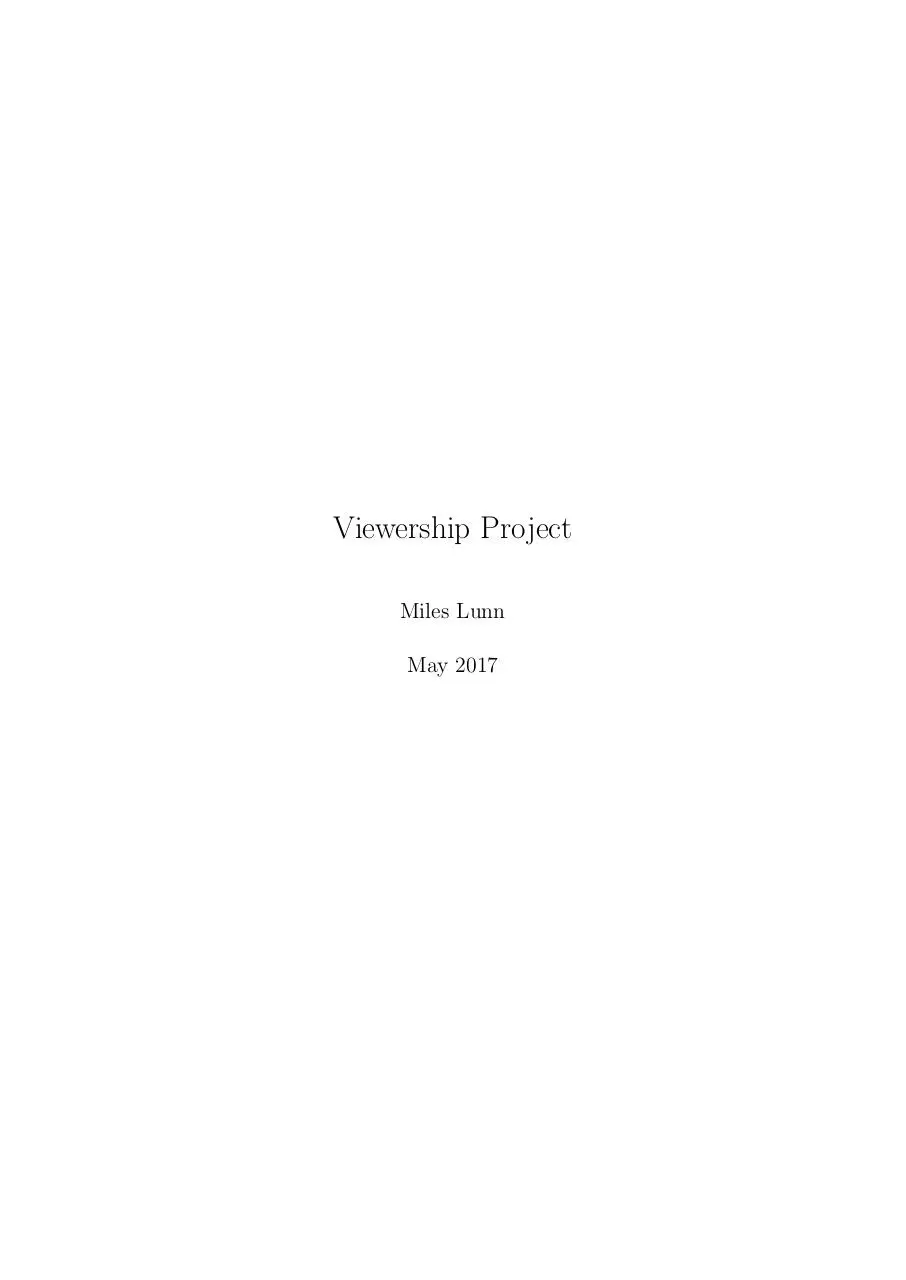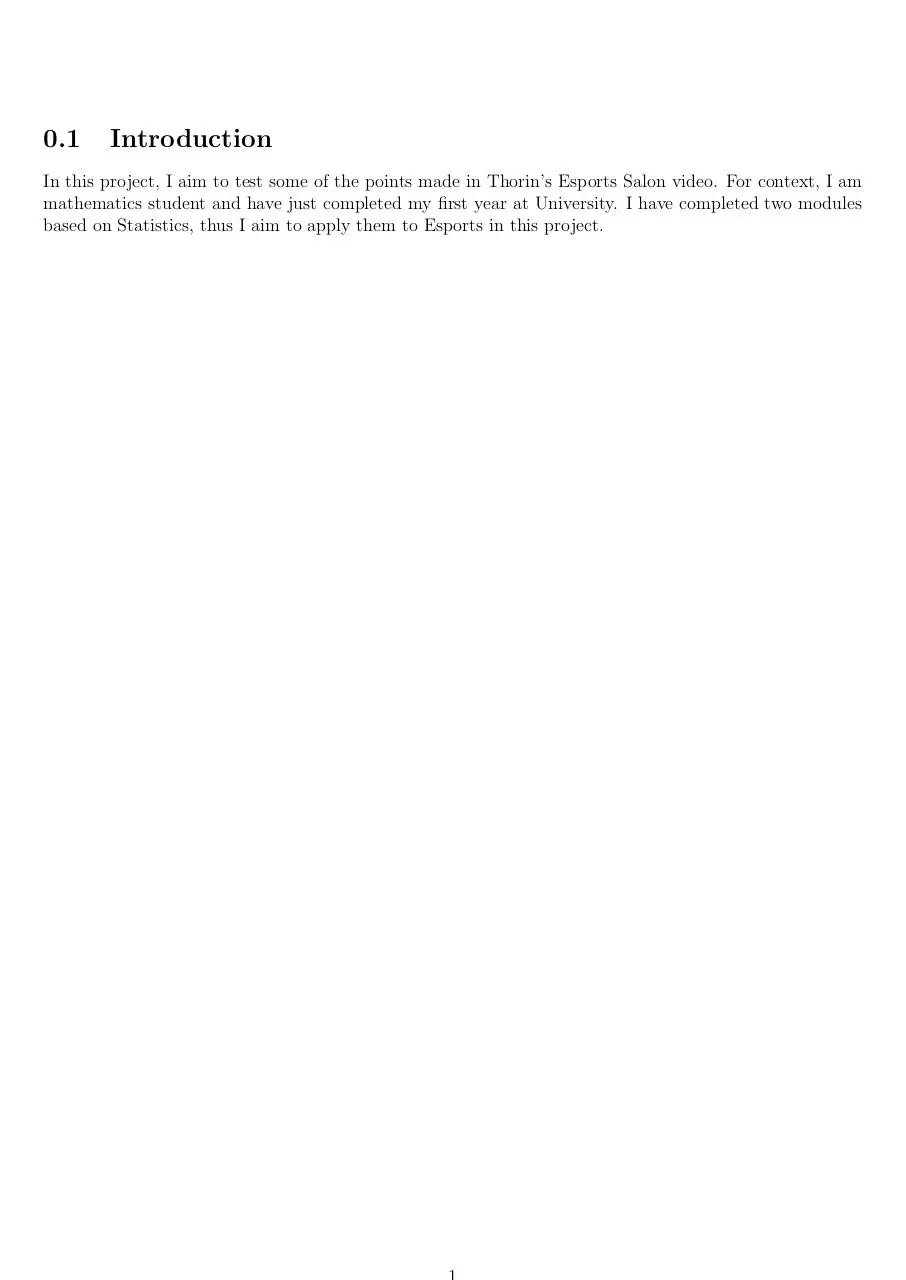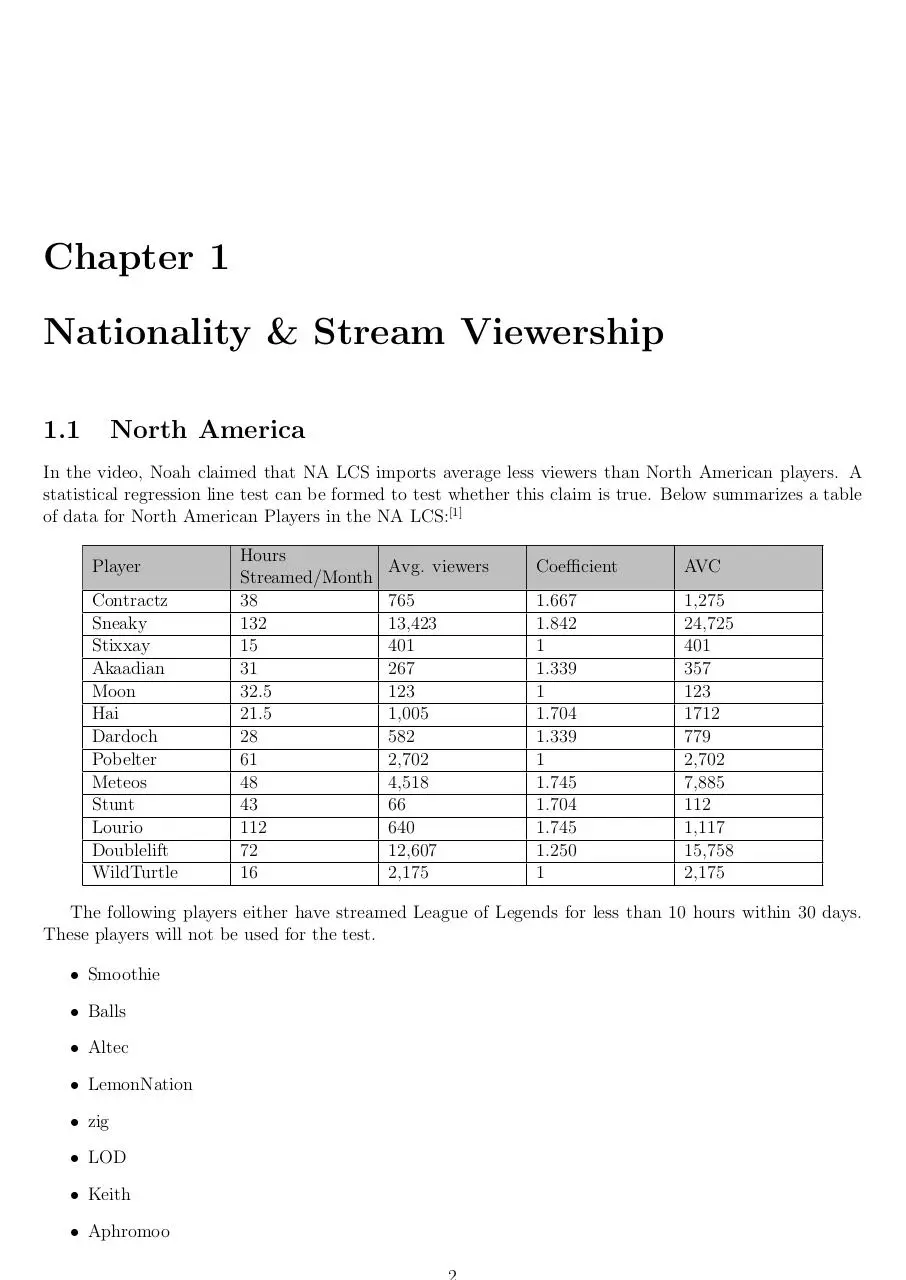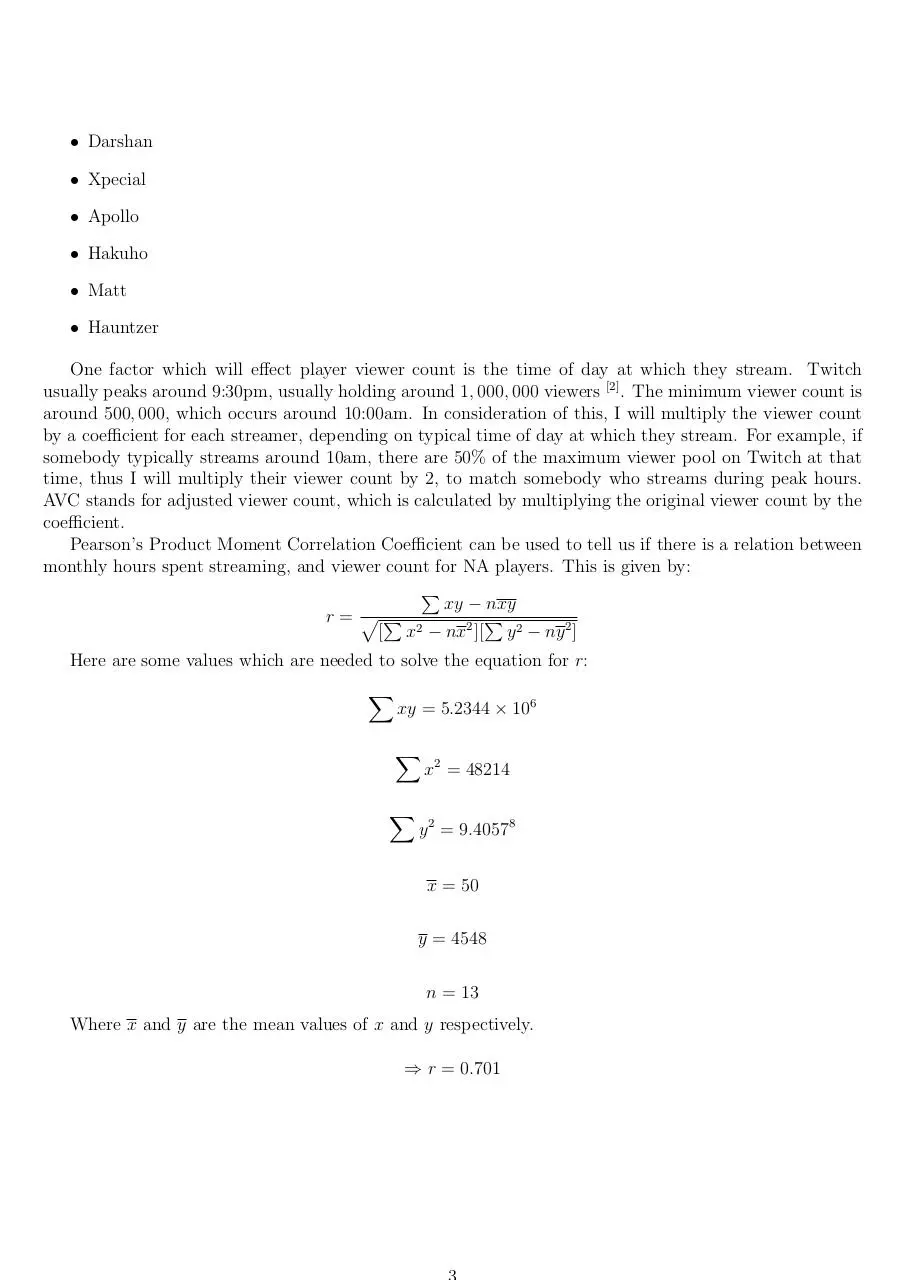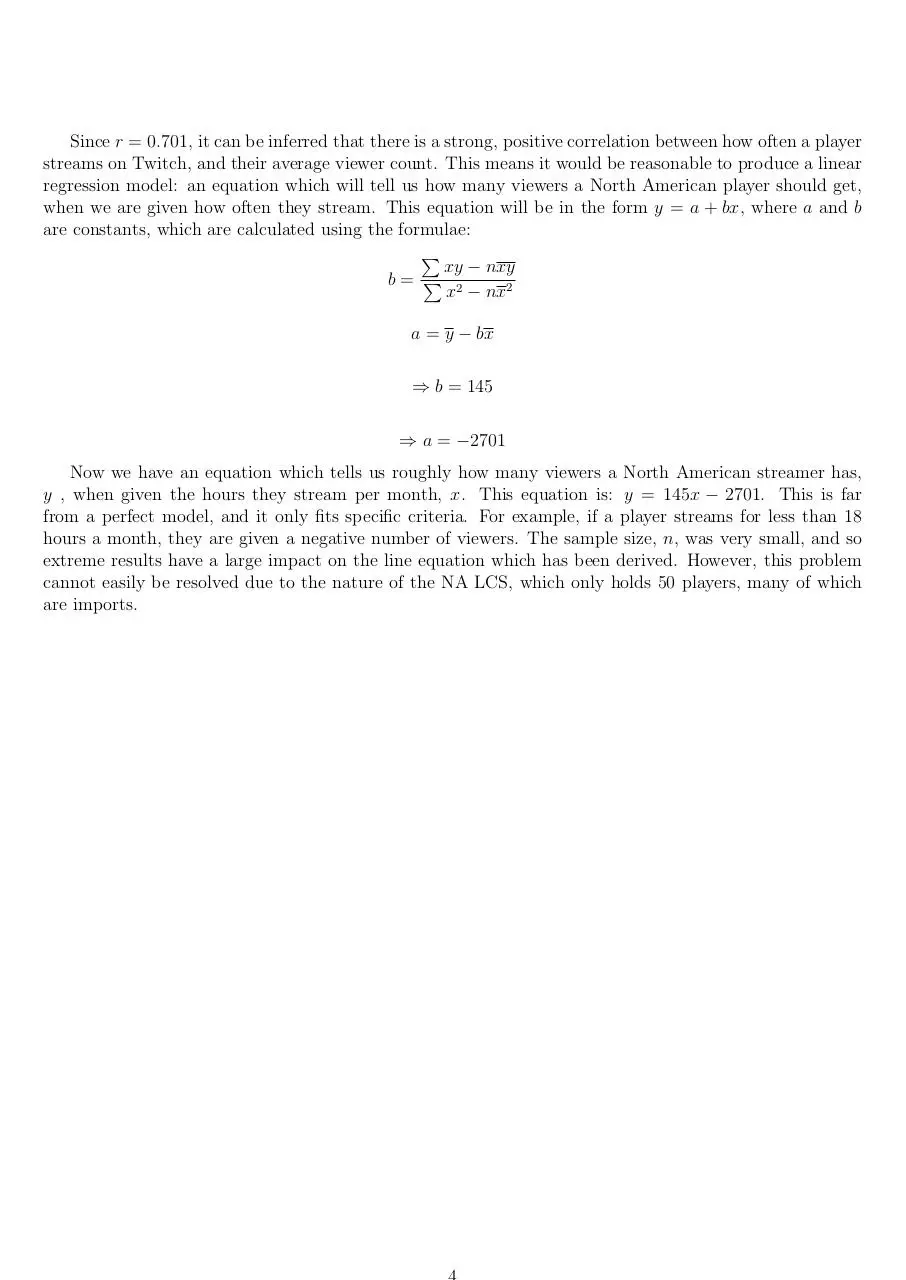ESS.pdf (PDF, 149.73 KB)

### Share on social networks

#### HTML Code

Copy the following HTML code to share your document on a Website or Blog